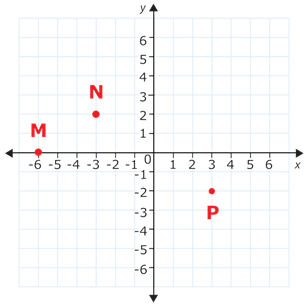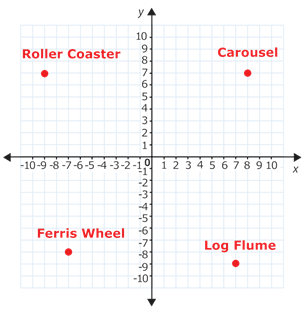# 4.1.2: Points in the Coordinate Plane

•• Contributed by CK12
• CK12

## Points in the Coordinate Plane

Megan is on vacation in Rome and is trying to find the restaurant where she will meet her friends for lunch. She got a map from her hotel that is formatted like a coordinate grid. The hotel is at the origin of the map and she was told that the restaurant is at the point (4,5) on the map. How can Megan find this location on the map so she can make it to the restaurant?

In this concept, you will learn how to name and graph ordered pairs of integer coordinates in a coordinate plane.

### Naming and Graphing Points in the Coordinate Plane

The coordinate plane is a grid created by a horizontal number line, the x-axis, intersecting a vertical number line, the y-axis. The point of intersection is called the origin.

The coordinate plane allows you to describe locations in two-dimensional space.

Each point on the coordinate plane can be named by a pair of numbers called an ordered pair in the form (x,y).

• The first number in an ordered pair identifies the x-coordinate of the point. This coordinate describes how far to the right or left of the y-axis a point is.
• The second number in an ordered pair identifies the y-coordinate of the point. This coordinate describes how far above or below the x-axis a point is.

Here is an example.

Plot the point (3,−4) on the coordinate plane.

This point has an x-coordinate of 3 and a y-coordinate of -4.

To plot this point, first start at the origin.

Then, find the location of the x-coordinate. Because the x-coordinate is positive, you will be moving to the right. Move to the right along the x-axis 3 units until you find 3.

Next, look at your y-coordinate. Because the y-coordinate is negative, you will be moving down. Move down from the 3 on the x-axis 4 units until you are lined up with the -4 on the y-axis.

The answer is shown plotted on the coordinate plane below.

Here is another example.

Give the ordered pair for the point plotted below.

To write the ordered pair you need both the x-coordinate and the y-coordinate.

Start at the origin. You need to figure out how far to the right/left you need to move and then how far up/down you need to move to reach your point.

First notice that you need to move 5 units to the right from the origin to reach the point. This means the x-coordinate is 5.

Next notice that you do not need to move up or down from the x-axis at all to reach the point. This means the y-coordinate is 0.

The answer is that the ordered pair is (5,0).

### Examples

Example 4.1.2.1

Earlier, you were given a problem about Megan, who is on vacation in Rome.

She's at her hotel and trying to use a map to find the location of the restaurant where she will be meeting her friends for lunch. The restaurant is at the point (4,5) on the map.

Solution

This point has an x-coordinate of 4 and a y-coordinate of 5.

To find this point, Megan should start at the origin on the map (her hotel).

Then, she should find the location of the x-coordinate. Because the x-coordinate is positive, she will be moving to the right. She needs to move to the right 4 units until she reaches the 4 on the x-axis.

Next, Megan should look at her y-coordinate. Because the y-coordinate is positive, she will be moving up. She should move up 5 units from the 4 on the x-axis.

The answer is shown plotted on the coordinate plane below.

Example 4.1.2.2

This coordinate grid shows locations in Jimmy’s city. Name the ordered pair that represents the location of the city park.

Solution

To write the ordered pair you need both the x-coordinate and the y-coordinate.

Start at the origin. You need to figure out how far to the right/left you need to move and then how far up/down you need to move to reach your point.

First notice that you need to move 2 units to the left from the origin in order to be exactly above the point for the city park. This means the x-coordinate is -2.

Next notice that you need to move down 6 units from the -2 on the x-axis to reach the point. This means that the y-coordinate is -6.

The arrows below show how you should have moved your finger to find the coordinates

The answer is that the ordered pair for the city park is (−2,6).

Example 4.1.2.3

Plot the point (0,3) on the coordinate plane.

Solution

This point has an x-coordinate of 0 and a y-coordinate of 3.

To plot this point, first start at the origin.

Then, find the location of the x-coordinate. Because the x-coordinate is 0, you do not need to move to the right or to the left from the origin. Stay at the origin.

Next, look at your y-coordinate. Because the y-coordinate is positive you will be moving up. Move up from the origin 3 units until you are lined up with the 3 on the y-axis.

The answer is shown plotted on the coordinate plane below.

Example 4.1.2.4

Give the ordered pair for the point plotted below.

Solution

To write the ordered pair you need both the x-coordinate and the y-coordinate.

Start at the origin. You need to figure out how far to the right/left you need to move and then how far up/down you need to move to reach your point.

First notice that you need to move 6 units to the right from the origin to be exactly above the point. This means the x-coordinate is 6.

Next notice that you need to move down 3 units from the 6 on the x-axis to reach your point. This means your y-coordinate is -3.

The answer is that the ordered pair is (6,−3).

Example 4.1.2.5

Plot the point (−2,−5) on the coordinate plane.

Solution

This point has an x-coordinate of -2 and a y-coordinate of -5.

To plot this point, first start at the origin.

Then, find the location of the x-coordinate. Because the x-coordinate is negative, you will be moving to the left. Move to the left 2 units until you reach the -2 on the x-axis.

Next, look at your y-coordinate. Because the y-coordinate is negative, you will be moving down. Move down 5 units from the -2 on the x-axis.

The answer is shown plotted on the coordinate plane below.

### Review

1. Name the ordered pair that represents each of these points on the coordinate plane.Figure 4.1.2.11 Below is a map of an amusement park. Name the ordered pair that represents the location of each of these rides.Figure 4.1.2.12
1. roller coaster
2. Ferris wheel
3. carousel
4. log flume

Name the ordered pairs that represent the vertices of triangle FGH.

1. F
2. G
3. H

Name the ordered pairs that represent the vertices of pentagon ABCDE.

1. A
2. B
3. C
4. D
5. E
6. On the grid below, plot point V at (−6,4).
1. On the grid below, plot point a triangle with vertices R(4,−1),S(4,−4) and T(−3,−4).

### Vocabulary

Term Definition
x−axis The x−axis is the horizontal axis in the coordinate plane, commonly representing the value of the input or independent variable.
x−coordinate The x−coordinate is the first term in a coordinate pair, commonly representing the value of the input or independent variable.
y−axis The y−axis is the vertical number line of the Cartesian plane.
Abscissa The abscissa is the x−coordinate of the ordered pair that represents a plotted point on a Cartesian plane. For the point (3, 7), 3 is the abscissa.
Cartesian Plane The Cartesian plane is a grid formed by a horizontal number line and a vertical number line that cross at the (0, 0) point, called the origin.
Coordinate Plane The coordinate plane is a grid formed by a horizontal number line and a vertical number line that cross at the (0, 0) point, called the origin. The coordinate plane is also called a Cartesian Plane.
Ordinate The ordinate is the y-coordinate of the ordered pair that represents a plotted point on a Cartesian plane. For the point (3, 7), 7 is the ordinate.
Origin The origin is the point of intersection of the x and y axes on the Cartesian plane. The coordinates of the origin are (0, 0).